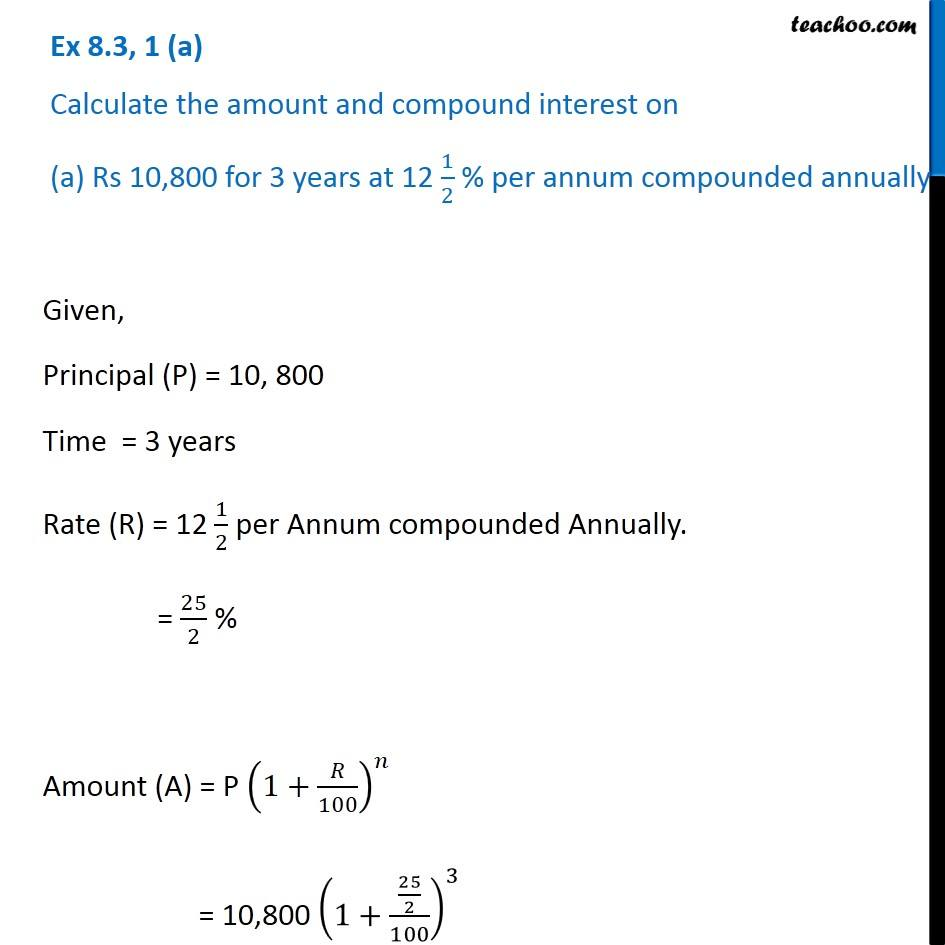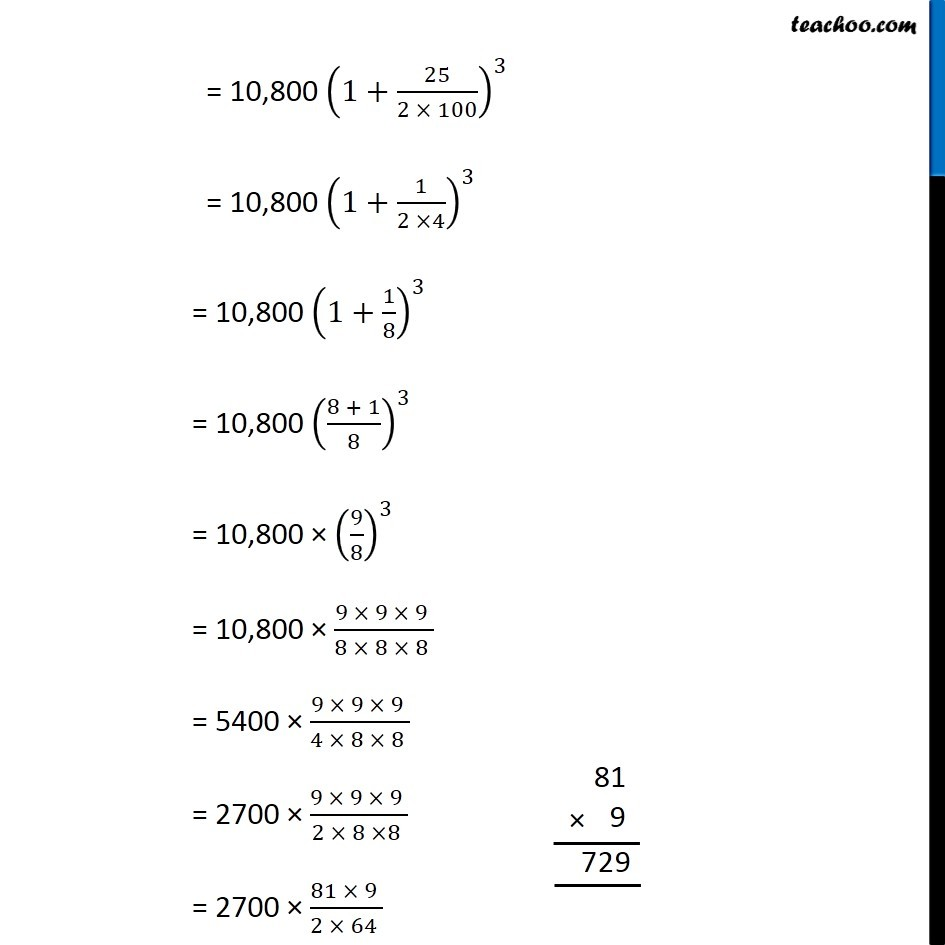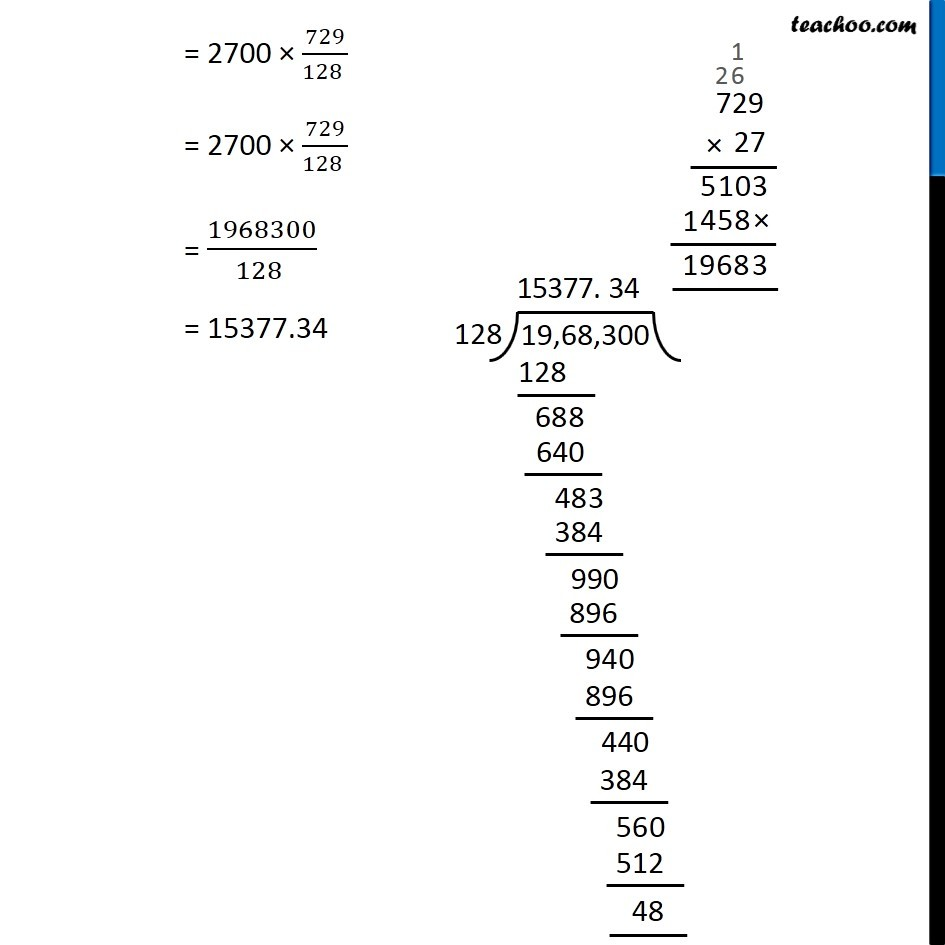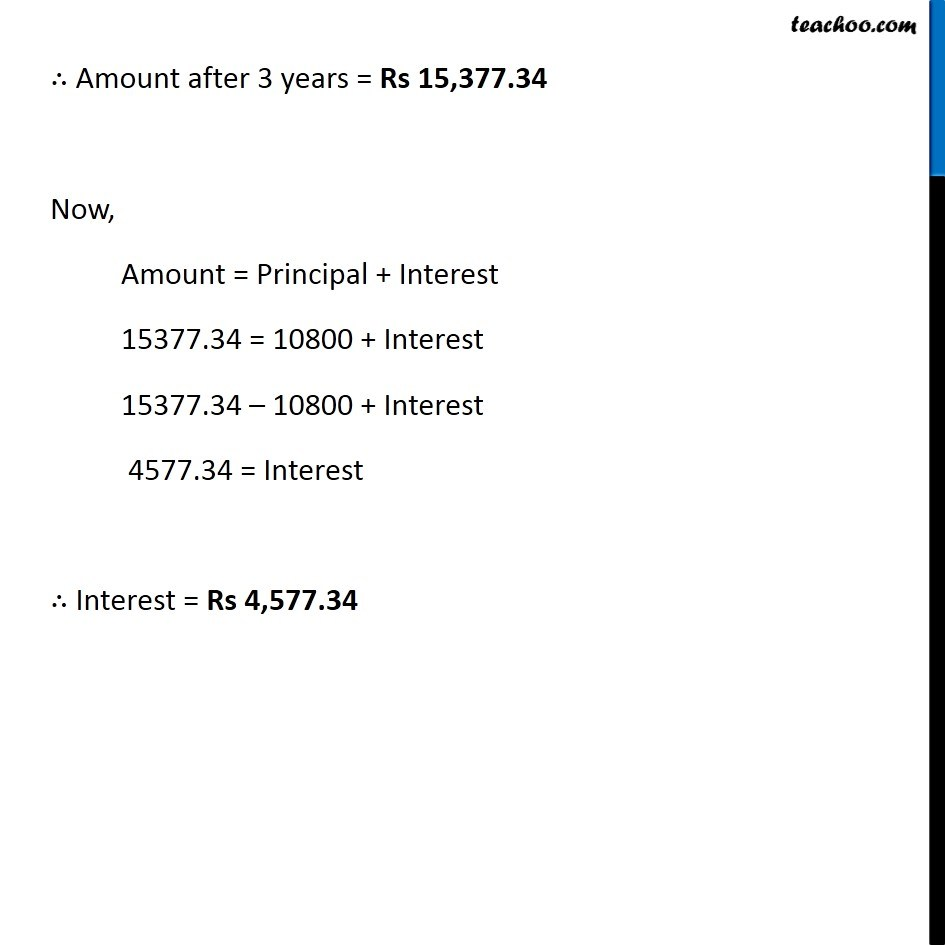Ex 7.3

Chapter 7 Class 8 Comparing Quantities
Serial order wiseLearn in your speed, with individual attention - Teachoo Maths 1-on-1 Class

### Transcript

Question 1 1 (a) Calculate the amount and compound interest on (a) Rs 10,800 for 3 years at 12 1/2 % per annum compounded annually Given, Principal (P) = 10, 800 Time = 3 years Rate (R) = 12 1/2 per Annum compounded Annually. = 25/2 % Amount (A) = P (1+𝑅/100)^𝑛 = 10,800 (1+(25/2)/100)^3 = 10,800 (1+25/(2 × 100))^3 = 10,800 (1+1/(2 ×4))^3 = 10,800 (1+1/8)^3 = 10,800 ((8 + 1)/8)^3 = 10,800 × (9/8)^3 = 10,800 × (9 × 9 × 9 )/(8 × 8 × 8 ) = 5400 × (9 × 9 × 9 )/(4 × 8 × 8 ) = 2700 × (9 × 9 × 9 )/(2 × 8 ×8 ) = 2700 × (81 × 9 )/(2 × 64 ) = 2700 × 729/(128 ) = 2700 × 729/(128 ) = 1968300/(128 ) = 15377.34 ∴ Amount after 3 years = Rs 15,377.34 Now, Amount = Principal + Interest 15377.34 = 10800 + Interest 15377.34 – 10800 + Interest 4577.34 = Interest ∴ Interest = Rs 4,577.34